Next: Effective Field Theory Up: FEW-NUCLEON SYSTEMS Previous: Nucleon-Nucleon Interaction

## The Deuteron, and more about the Dynamical Symmetry Breaking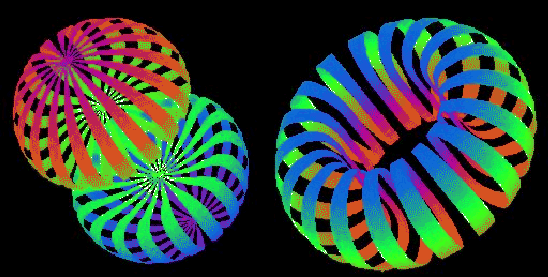Having defined the two-body force that acts between the nucleons, we can relatively easily find the ground-state wave function of the deuteron, and calculate all its properties. In doing so one cannot forget that for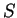=1 states, the tensor terms in the interaction can mix interaction channels, i.e., for any angular momentum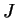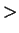0, states with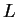=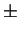1 are mixed if their parity equals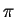=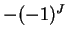. These conditions are fulfilled for the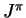=1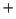deuteron ground state, and hence interactions channels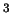S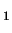andDcontribute to the deuteron ground-state wave function.

The solution corresponding to the Argonne v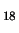interaction is illustrated in Fig. 6, where surfaces of equal density are shown for the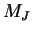=0 and 1 magnetic substates of the=1 deuteron ground state. Interested students are invited to visit the WEB site indicated in the Figure caption, to see the animation that shows similar surfaces at other densities. The surfaces are here shown by stripes that allow seeing the other side of the deuteron. The colors are used only to enhance the three-dimensional rendition of the image, and have no other meaning. In particular, the fact that the front piece of the left part in Fig. 6 is red, and the rear piece is blue, does not mean that the neutron is represented in red and the proton in blue, nor that it has been rendered the other way around. In reality, the laboratory-frame wave function has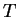=0, i.e, it is an antisymmetrized combination of products of the neutron and proton wave functions.

This brings us to a very important point pertaining to the dynamical (or spontaneous) symmetry breaking mechanism discussed already in Sec. 2.5. Suppose that you are confronted with a request: S'il vous plaît...dessine-moi un deuton! (see Ref. Exu96 for an analogous example). Without any deep information about the interaction, you would draw to points (or spheres, if you know something about quantum mechanics), some distance apart, and mark one of them with a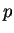and the other one with an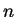. And this is what the deuteron really looks like in the so-called intrinsic reference frame.

One should not attribute too much importance to the descriptions laboratory frame'' and intrinsic frame''. Below we shall use this names at will, but let us rather treat them as proper names describing two different ways of constructing the wave functions, and not as mathematically sound representations of the same wave function in two different reference frames.

The intrinsic wave function of the deuteron breaks the rotational symmetry, and breaks the isospin symmetry, i.e., a rotation in the real space, and a rotation in the iso-space, gives another wave function. In a more mathematical language, such a wave function does not belong to any single representation of the rotational and isospin symmetry groups. You should not be confused by the fact that the laboratory-frame=1 wave function has three magnetic components (two of them are illustrated in Fig. 6), and hence none of them is strictly invariant with respect to the real-space rotations. However, each magnetic component, when rotated, is equal to some linear combination of all magnetic components, i.e., the=1 state is invariant with respect to rotations in this more general sense - it belongs to one, single representation of the rotation group.

Before discussing the sense of the intrinsic wave functions, let us give two other examples of the symmetry-broken intrinsic wave functions. Imagine the ground-state wave function of the water molecule H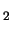O. We know very well how this molecule looks like - the two hydrogen atoms are connected by chemical bonds to the oxygen atom, and the two lines connecting the H and O nuclei form an angle of about 105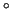. So the wave function of the water molecule breaks the rotational invariance. However, if we take such an isolated molecule, and wait long enough for all its rotational and vibrational excitations to de-excite by the emission of electromagnetic radiation, the molecule will reach the ground state of=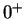, i.e., the state which is perfectly invariant with respect to rotations.

There is no contradiction between these two pictures of the molecule. The first one pertains to the wave function in the intrinsic reference frame, and the second one to the wave function in the laboratory reference frame. The intrinsic wave function is not an exact ground state of the rotationally invariant Hamiltonian. It is a wave packet, which has a good orientation in space, and a very broad distribution of different angular momenta, corresponding to the ground-state rotational band of the water molecule. On the contrary, the laboratory-frame wave function is an exact ground state of the rotationally invariant Hamiltonian, it has a definite value of the angular momentum,=0, and has a completely undefined orientation in space.

As the second example, consider the ground state of the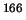Er nucleus. It is a well-deformed nucleus, having the intrinsic ground-state wave function in the form of a cigar (prolate shape), which breaks the rotational symmetry. At the same time, the laboratory ground state has=, and is perfectly rotationally invariant. Again, the cigar-shape, intrinsic wave function is a wave packet that is oriented in space and has an undefined angular momentum, while the laboratory wave function is an exact eigenstate having a definite angular momentum.

Now comes a very important question, namely, is there anything else in the phenomenon of the dynamical symmetry breaking apart from the trivial wave-packet formation? The answer is, of course, yes! The point is that some systems can, and some other ones cannot be oriented. The first ones do break the symmetry dynamically, and the second ones do not. It is obvious that the water molecule does it. In other words, its moment of inertia is so huge that the ground-state rotational band is very much compressed (compared to other possible excitations), and all rotational states of this band (all different angular momenta) are very close to one another. The wave packet built of such states is therefore almost'' an eigenstate - at least it has a very long lifetime before it decays to the ground state. Hence, the oriented state of the water molecule is a very good rendition of the exact ground state.

On a different scale, the same is true for theEr nucleus. States of its ground-state rotational band live some nanoseconds, i.e., much longer that any other excitations available in this system. Hence, this nucleus can be oriented, and the corresponding wave packet fairly well represents the ground state. This representation is better or worse depending on which observable we want to look at. For example, if we measure the nuclear root-mean-square radius, the oriented wave function can be used at marvel. The increase of radii of deformed nuclei as compared to their spherical neighbours is a very well established experimental fact. Similarly, lifetimes of the rotational states can be very well approximated by the probability of emitting classical radiation from a rotating charged deformed body.

So we can really say that the ground-state=wave function ofEr does break, and that of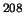Pb does not dynamically break the rotational symmetry. The latter nucleus does not have any rotational band and thus the oriented wave packet cannot exist. Both=ground-state wave functions are perfectly rotationally invariant, while the dynamical symmetry breaking is a notion pertaining to their intrinsic structure.

The utility of the intrinsic wave function does not end at systems that dynamically break the symmetry. Namely, often it is very easy to construct approximated symmetry-broken wave functions, and then use its symmetry-projected component to model the exact symmetry-invariant ground state. The deuteron wave function, with which we have begun this discussion, is a perfect example of such a situation. Namely, the intrinsic-frame image of this nucleus (neutron here and proton there) breaks the isospin symmetry, but the component projected on=0 is a very good representation of the exact wave function. In this case, projection on=0 simply means antisymmetrizing the two components with the neutron and proton positions exchanged. The=0 projected component serves us well, even if the=1 component (=0) is unbound at all.

Moreover, the intrinsic-frame image of the deuteron explains very well why this particle has apparently so different shapes depending on the value of the magnetic projection. The=0, torus-like shape, Fig. 6, results simply from projecting the intrinsic wave function on=1 and=0, which corresponds to taking a linear superposition of all intrinsic states rotated around the axis perpendicular to the line connecting the neutron and proton in the intrinsic frame. Without such an interpretation, nobody would actually believe that deuteron looks like a torus.Next: Effective Field Theory Up: FEW-NUCLEON SYSTEMS Previous: Nucleon-Nucleon Interaction
Jacek Dobaczewski 2003-01-27